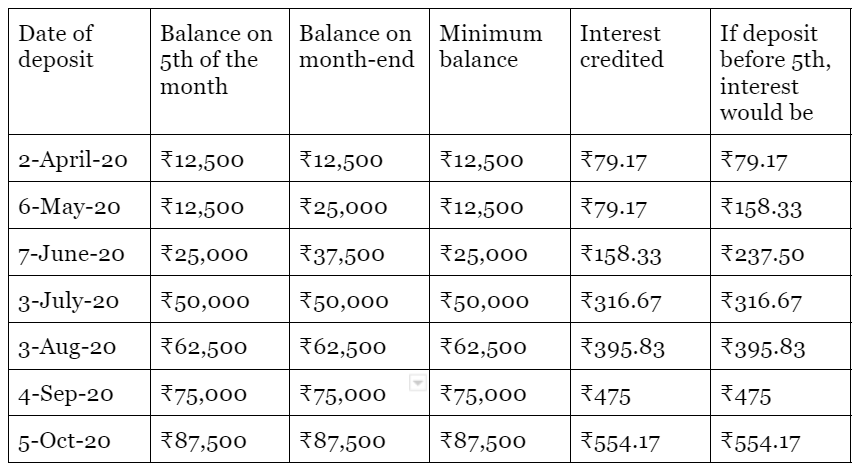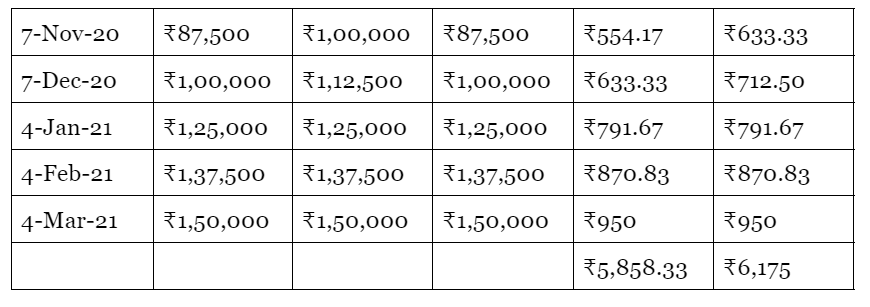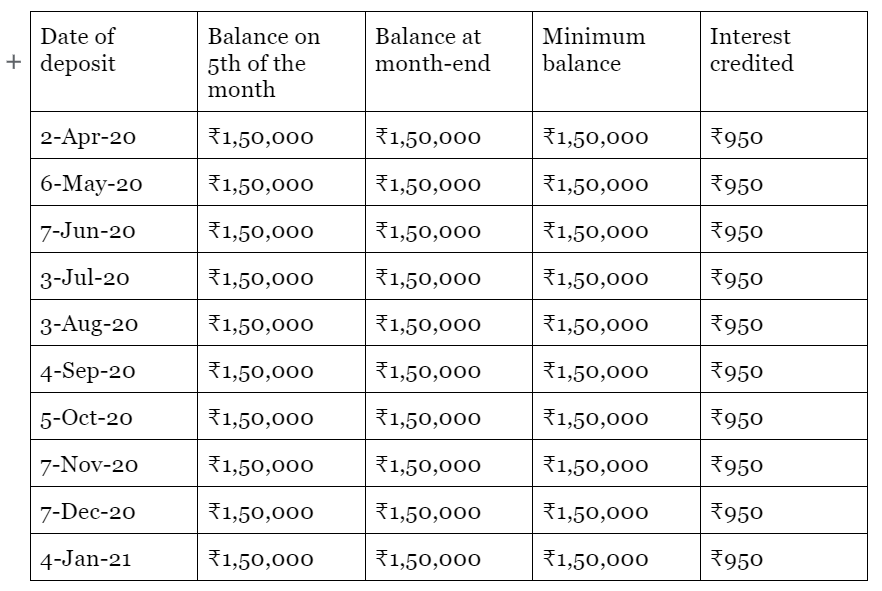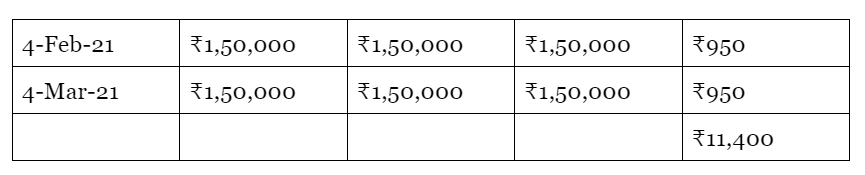CREDcalculatorsarticles
Is PPF a good investment tool for you? find out here

# Is PPF a good investment tool for you? find out here

finance
July 8, 2022The public provident fund (PPF) is one of the best investment options that not only offer the tax benefits under Section 80C of the Income Tax Act (old tax regime) but also gives the tax-free interest and maturity benefit.

The Indian government reviews and releases the applicable interest rate on PPF investment every quarter. as per the latest update, the PPF interest rate for the quarter ending June 30, 2021, is 7.1 percent per annum (compounded yearly).

As per the public provident fund rules, the interest on your PPF account is calculated on a monthly basis but the amount is credited to your account at the end of the financial year on March 31. However, note that the interest becomes payable for that month only if the PPF contribution is made before the fifth of that month.

You can invest a minimum of ₹500 and a maximum of ₹1.5 lakh in a financial year in a PPF account. The public provident fund accounts have a maturity period of 15 years.

formula used to calculate interest on PPF investment

PPF account interest is calculated on the minimum balance available in your PPF account between the 5th and the end of each month. If you deposit your PPF contribution before the 5th of each month, you will get the interest for that month on that contribution, otherwise, your PPF account interest will be calculated on the previous balance.

Therefore, to maximize PPF interest, financial experts recommend making PPF contributions or lump sum deposits before the 5th of each month. You can deposit a minimum of ₹500 and a maximum of ₹1.5 lakh in a PPF account in a year. Also, note that if you have opened a PPF account in your child's name with you as the guardian of the minor, the maximum amount that you can deposit in your account including your child’s account is ₹1.5 lakh per year.

If you have the fund, it's advised that you should deposit ₹1.5 lakh in a lump sum by the 5th of April to earn interest for the rest of the year.

The formula to calculate the interest on PPF account is provided below for reference:

f = p[({(1+i)^n}-1)/i]

where,

f is the maturity proceeds of the PPF

p is the annual installments

n is the number of years or tenure of investment

i is the rate of interest/100

Now let's see how interest on PPF investment is calculated in the case of lump-sum and monthly contributions:

Interest earned on PPF monthly contribution

You can deposit a maximum of ₹12,500 per month in a PPF account. So let's suppose you make a monthly contribution of ₹12,500 and the interest rate for PPF is 7.6 percent and remains constant throughout the financial year. So, here's how your PPF interest will be calculatedHere you can see that if you contributed before the 5th of every month, you would have earned ₹316.67 more in the financial year.

Interest earned on PPF lump-sum deposit

You can contribute a maximum of ₹1,50,000 to a PPF account in a financial year. Let’s see how the interest is calculated in this case:Here you see that if you would have made a lump sum contribution after the 5th of April, you would have not got the interest for April month, hence, your interest earned would have decreased to ₹10,450.

Therefore, in a PPF investment, it’s very important that you make your monthly contribution before the 5th of every month, and in the case of a lump sum, deposit the amount before the 5th of April to gain maximum interest benefit.

Online PPF calculator

Manual calculation of PPF interest can be lengthy and confusing for many. To make the PPF interest calculation easier and faster, you can use the CRED PPF calculator. It is a free calculator that will help you to estimate the interest and returns while you are planning your PPF investments.

Here's why you should use the CRED PPF calculator:

• CRED PPF calculator will make your calculations faster and easier.
• You can get clear data of how much interest you can earn on investing a certain amount.
• You can use the CRED PPF calculator anywhere, anytime.
• You can use the CRED PPF calculator over and over again until you achieve a clear balance between your investment amount and desired returns.
• Since the CRED PPF calculator is automated, you can avoid calculation errors.
• CRED PPF calculator can help you in investment and tax planning so that you can use your savings in a better way.
• You can use the CRED PPF calculator to figure out how much wealth you can grow until your retirement.

How to use the CRED PPF calculator?

The CRED PPF calculator is self-explanatory and simple to use. Here’s a step-by-step guide on how to use the CRED PPF calculator:

• Open the CRED website and click on calculators
• Click on the PPF calculator
• Select your PPF yearly investment amount
• Select the time period (tenure) of your PPF investment
• Select the rate of interest
• The CRED PPF calculator will instantly show the maturity value of your PPF investment
• You can also check your total investment for the selected tenure and total interest earned on your PPF investment

use CRED PPF calculator now